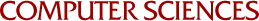## A Transitive Closure Algorithm

Paul W. Purdom
1968

An algorithm is given for computing the transitive closure of a directed graph in a time no greater than a1 N1 n + a2n2 for large n where a1 and a2 are constants depending on the computer used to execute the algorithm, n is the number of nodes in the graph and N1 is the number of arcs (not counting those arcs which are part of a cycle and not counting those arcs which can be removed without changing the transitive closure). For graphs where each arc is selected at random with probability p , the average time to compute the transitive closure is no greater than min {a1 pn3 + a2n2, 1/2a1n2p-2+a2n2} for large n . The algorithm will compute the transitive closure of an undirected graph in a time no greater than a2n2 for large n. The method uses about n2 + n bits and 5n words of storage (where each word can hold n + 2 values).